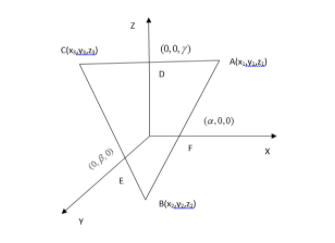QuestionAnswers

# A triangle ABC is placed so that the mid-points of the sides are on the x,y,z axes. Lengths of the intercepts made by the plane containing the triangle on these axes are respectively $\alpha ,\beta ,\gamma$. Coordinates of the centroid of the triangle ABC areA. $( - \alpha /3,\beta /3,\gamma /3)$B. $(\alpha /3, - \beta /3,\gamma /3)$C. $(\alpha /3,\beta /3, - \gamma /3)$D. $(\alpha /3,\beta /3,\gamma /3)$Verified
129k+ views
Hint: A triangle ABC is placed so that the mid-points of the sides are on the x,y,z axes. Lengths of the intercepts made by the plane containing the triangle on these axes are respectively$\alpha ,\beta ,\gamma$. Coordinates of the centroid of the triangle ABC are
$( - \alpha /3,\beta /3,\gamma /3)$
$(\alpha /3, - \beta /3,\gamma /3)$
$(\alpha /3,\beta /3, - \gamma /3)$
$(\alpha /3,\beta /3,\gamma /3)$

Complete step by step solution:
1) $\,ABC$ is placed so that the mid-points of the sides are on the x,y,z axes
i.e.:D,E,F are the mid-points of CA , BC , BA respectively and D,E,F lie on the Z,Y,X axes.
2) Lengths of the intercepts made by the plane containing the triangle on these axes are $\alpha ,\beta ,\gamma$respectively which means the coordinates of D,E,F are $F(\alpha ,0,0),E(0,\beta ,0)and\,D(0,0,\gamma )$.Here, $A = ({x_1},{y_1},{z_1})$
$B = ({x_2},{y_2},{z_2})$
$C = ({x_3},{y_3},{z_3})$
And $F = (\alpha ,0,0)$
$E = (0,\beta ,0)$
$D = (0,0,\gamma )$
Step 1: To make a relation between the coordinates of A,B,C with $\alpha ,\beta ,\gamma$
i)= > $F = (\dfrac{{{x_1} + {x_2}}}{2},\dfrac{{{y_1} + {y_2}}}{2},\dfrac{{{z_1} + {z_2}}}{2})$ [ F is the mid point of AB]
= > $F = (\alpha ,0,0)$
i.e.: $\dfrac{{{x_1} + {x_2}}}{2} = \alpha ,\,\,\dfrac{{{y_1} + {y_2}}}{2} = 0,\,\,\dfrac{{{z_1} + {z_2}}}{2} = 0$
ii) = > $E = (\dfrac{{{x_2} + {x_3}}}{2},\dfrac{{{y_2} + {y_3}}}{2},\dfrac{{{z_2} + {z_3}}}{2})$ [ E is the mid point of BC]
= > $F = (0,\beta ,0)$
i.e.: $\dfrac{{{x_2} + {x_3}}}{2} = 0,\,\,\dfrac{{{y_2} + {y_3}}}{2} = \beta ,\,\,\dfrac{{{z_2} + {z_3}}}{2} = 0$
=> $D = (\dfrac{{{x_3} + {x_1}}}{2},\dfrac{{{y_3} + {y_1}}}{2},\dfrac{{{z_3} + {z_1}}}{2})$
= > $D = (0,0,\gamma )$
i.e.: $\dfrac{{{x_3} + {x_1}}}{2} = 0,\,\,\dfrac{{{y_3} + {y_1}}}{2} = 0,\,\,\dfrac{{{z_3} + {z_1}}}{2} = \gamma$
From (i) , We get,
${x_1} + {x_2} = 2\alpha$
${y_1} + {y_2} = 0$
${z_1} + {z_2} = 0$
From (ii), we get,
${x_2} + {x_3} = 0$
${y_2} + {y_3} = 2\beta$
${z_2} + {z_3} = 0$
From (iii), we get,
${x_3} + {x_1} = 0$
${y_3} + {y_1} = 0$
${z_3} + {z_1} = 2\gamma$
From all the above equations, we can conclude that,
${x_1} + {x_2} + {x_3} = \alpha$
${y_1} + {y_2} + {y_3} = \beta$
${z_1} + {z_2} + {z_3} = \gamma$
Hence, we get the coordinates of the points A,B,C in terms of $\alpha ,\beta ,\gamma$
Step 2: We will now find out the coordinate of centroid of the triangle coordinate of the centroid of the triangle is given by
$(\dfrac{{{x_1} + {x_2} + {x_3}}}{3},\dfrac{{{y_1} + {y_2} + {y_3}}}{3},\dfrac{{{z_1} + {z_2} + {z_3}}}{3})$
= $(\dfrac{\alpha }{3},\dfrac{\beta }{3},\dfrac{\gamma }{3})$
Hence, the coordinate of the centroid of the triangle ABC is $(\dfrac{\alpha }{3},\dfrac{\beta }{3},\dfrac{\gamma }{3})$.
So, $D(\dfrac{\alpha }{3},\dfrac{\beta }{3},\dfrac{\gamma }{3})$ is the correct answer.

Note: Diagram should be drawn properly. As because, a correct diagram will only lead you to a correct answer. Also, equations should be written correctly.